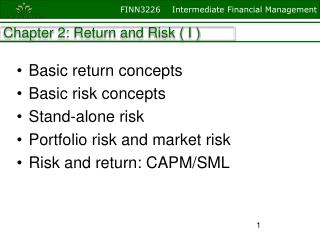Download PresentationChapter 2: Return and Risk ( I )

Chapter 2: Return and Risk ( I ) - PowerPoint PPT Presentation

Chapter 2: Return and Risk ( I ). Basic return concepts Basic risk concepts Stand-alone risk Portfolio risk and market risk Risk and return: CAPM/SML . 1. What are investment returns?. Investment returns measure the financial results of an investment.I am the owner, or an agent authorized to act on behalf of the owner, of the copyrighted work described.
Download PresentationChapter 2: Return and Risk ( I )

Download Policy: Content on the Website is provided to you AS IS for your information and personal use and may not be sold / licensed / shared on other websites without getting consent from its author.While downloading, if for some reason you are not able to download a presentation, the publisher may have deleted the file from their server.

- - - - - - - - - - - - - - - - - - - - - - - - - - E N D - - - - - - - - - - - - - - - - - - - - - - - - - -
Presentation Transcript
1. Chapter 2: Return and Risk ( I ) • Basic return concepts • Basic risk concepts • Stand-alone risk • Portfolio risk and market risk • Risk and return: CAPM/SML 1

2. What are investment returns? • Investment returns measure the financial results of an investment. • Returns may be historical or prospective (anticipated). • Returns can be expressed in: • Dollar terms. • Percentage terms.

3. Realized Return An investment costs \$1,000 and is sold after 1 year for \$1,100: Dollar return: \$ Received - \$ Invested \$1,100 - \$1,000 = \$100. Percentage return: \$ Return/\$ Invested \$100/\$1,000 = 0.10 = 10%.

4. Expected Return • Expected Return is the weighted average of all the possible returns, weighted by the probability that each return will occur. • Expected Return (%) = ΣPbi*ri • Where Pbi = probabilities of outcome i • ri = expected % return in outcome

5. What is investment risk? • Typically, investment returns are not known with certainty. • Investment risk pertains to the probability of earning a return less than that expected. • The greater the chance of a return far below the expected return, the greater the risk.

6. What is Investment Risk? Probability Distribution: Which stock is riskier? Why?

7. Standard Deviation (S.D.) • Standard deviation (S.D.) is one way to measure risk. It measures the volatility or riskiness of portfolio returns. • S.D. = square root of the weighted average squared deviation of each possible return from the expected return.

8. Standard Deviation (S.D.)

9. Risk Measure For Basic Foods, S.D.=? 10

10. Stand-Alone Risk • Standard deviation measures the stand-alone risk of an investment. • The larger the standard deviation, the higher the probability that returns will be far below the expected return. • So which one of sale.com and basic foods is high risky? • But what if this case?

11. Measuring Stand–Alone Risk: The Coefficient of Variation How do we choose between two investments if one has a higher expected return and the other a lower standard deviation? To help answer this question, we often use another measure of risk, the coefficient of variation (CV) , which is the standard deviation divided by the expected return: CV = Standard deviation / expected return CVS-Stock = 32.6% / 16.7% = 1.9521 CVL-Stock = 20.4% / 11.9% = 1.7143 CVT-BILLS = 3.1% / 3.7% =0.8378 12

12. Portfolio Return and Risk • Portfolio return rpis the weighted average of the expected returns on the individual assets in the portfolio. Suppose there are n stocks. The expected return on Stock i isri . The fraction of the portfolio's dollar value invested in Stock i (that is, the value of the investment in Stock i divided by the total value of the portfolio) is wi, and all the wi must sum to 1.0. The expected return on the portfolio is 13

13. Portfolio Return and Risk

14. ^ rp = (3.0%)*0.10 + (6.4%)*0.20 + (10.0%)*0.40 + (12.5%)*0.20 + (15.0%)*0.10 = 9.6% p = [(3.0 - 9.6)2*0.10 + (6.4 - 9.6)2*0.20 +(10.0 - 9.6)2*0.40 + (12.5 - 9.6)2*0.20 + (15.0 - 9.6)2*0.10]1/2 = 3.3% CVp = 3.3%/9.6% = .34 Portfolio Return and Risk

15. Portfolio vs. Its Components • Portfolio expected return (9.6%) is between stock A (17.4%) and stock B (1.7%) returns. • Portfolio standard deviation is much lower than: • either stock (20% and 13.4%). • average of stock A and stock B (16.7%). • The reason is due to negative correlation (r) between stock A and stock B returns.

16. Conclusion Stand-alone risk = Market risk + Diversifiable risk: • Market risk is that part of a security’s stand-alone risk that cannot be eliminated by diversification. • Firm-specific, or diversifiable, risk is that part of a security’s stand-alone risk that can be eliminated by diversification.

17. Beta and Systematic Risk CAPM suggests that Beta is a factor in determining the relation between returns and systematic risk. Market Risk Premium 19

18. How is market risk measured for individual securities? • Market risk, which is relevant for stocks held in well-diversified portfolios, is defined as the contribution of a security to the overall riskiness of the portfolio. • It is measured by a stock’s beta coefficient. For stock i, its beta is:

19. Portfolio Beta • An important aspect of the CAPM is that the beta of a portfolio is a weighted average of its individual securities' betas: Calculate beta for a portfolio with 50% Stock H and 50% Stock L: bp = Weighted average= 0.5(bStock H) + 0.5(bStock L) = 0.5*2 + 0.5*0.5 = 1.25 22